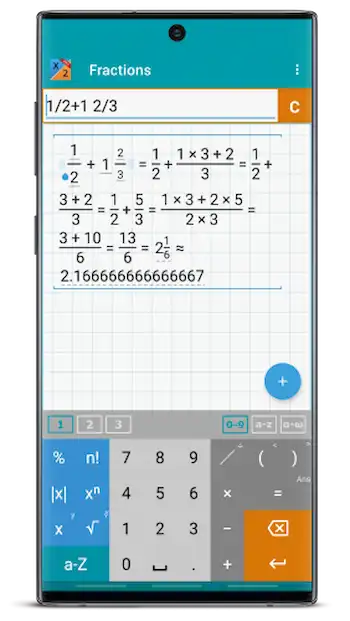# Fraction Calculator + Math PRO Patched MOD APK

#### 2022.50

• Payment
Fraction Calculator + Math PRO with step-by-step operations and algebra. Shows results as you type. Graphical display of expressions with history. Use space to enter the mixed numbers, e.g.
Developer
Mathlab Apps LLC
Updated
October 21, 2022
Size
8M
Version
2022.50
Requirements
5.0 and up

## Description

Fraction Calculator + Math PRO with step-by-step operations and algebra. Shows results as you type. Graphical display of expressions with history. Use space to enter the mixed numbers, e.g.## PRO FEATURES

* Workspaces to work on multiple tasks
* Store often-used expressions in a library

## FRACTION CALCULATOR

* Step-by-step operations (option to turn off)
* Arithmetic operations (+,-,*,/,÷), hold / to enter ÷
* Powers of fractions
* Fractions simplification
* Fractions with complex numbers
* Decimal to fraction conversion and back
* Symbolical fractions and operations
* Integer roots simplification
* Pinch to zoom

## ALGEBRA

* Linear equations x+1=2 -> x=1
* Quadratic equations x^2-1=0 -> x=-1,1
* Approximate roots of higher polynomials
* Systems of linear equations, write one equation per line, x1+x2=1, x1-x2=2
* Polynomial long division
* Polynomial expansion, factoring
* Solving inequalities with one variable.
Double tap “(“to enter the “less” sign.
Double tap “)” to enter the “greater” sign.
* Linear and polynomial inequalities, x^3-4>4
* Inequalities with absolute values, abs(2x+3)<=5
* Compound inequalities, 1* Rational inequalities, (x+3)/(x-1)<=0

## What's new

Day/night support

## Mod Info

• No root or Lucky Patcher or Google Play Modded required;
• Disabled / Removed unwanted Permissions + Receivers + Providers + Services;
• Optimized and zipaligned graphics and cleaned resources for fast load;
• Ads Permissions / Services / Providers removed from Android.manifest;